### 8. ENERGY CONVERSION

The cross section for a direct electromagnetic or nuclear interaction between the relativistic particles and the ISM particles is too small to convert efficiently the kinetic energy to radiation. The fireball particles can be slowed down only via some collective interaction such as a collisionless shock. Supernova remnants (SNRs) in which the supernova ejecta is slowed down by the ISM show that collisionless shocks form in somewhat similar circumstances. One can expect that collisionless shocks will form here as well [27, 18].

GRBs are the relativistic analogues of SNRs. In both cases the phenomenon results from the conversion of the kinetic energy of the ejected matter to radiation. Even the total energy involved is comparable. One crucial difference is the amount of ejected mass. SNRs involve a solar mass or more. The corresponding velocity is several thousands kilometers per second, much less than the speed of light. In GRBs, the masses are smaller by several orders of magnitude and with the same energy the matter attains ultra-relativistic velocities. A second crucial difference is that while SNRs result from the interaction of the ejecta with the ISM, GRBs result from internal collisions. The interaction of the ejecta in GRBs with the ISM produces the "afterglow" that follows some GRBs.

The interaction between the SNR ejecta and the ISM takes place on scales of several pc and it is observed for thousands of years. The internal interaction of the relativistic matter in GRBs takes place on a scale of several hundred astronomical units and special relativistic effects reduce the observed time scale to a fraction of a second. The interaction with the ISM that leads to the "afterglow" takes place on a scale of a tenth of a pc. Once more special relativistic effects reduce the observed time scale to several days.

In the following sections I discuss the slowing down of matter due to relativistic shocks. The discussion begins with a general review of relativistic inelastic collisions and continues with the relativistic shock conditions. Then I turn to the radiation processes: synchrotron emission and Inverse Compton. After the general discussion I apply the general results to internal shocks, to external shocks and to the afterglow.

Consider a mass (denoted by the subscript r) that catches up a slower one (denoted s). The two masses collide and merge to form a single mass (denoted m). Energy and momentum conservation yield: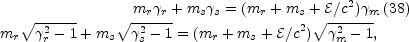(38)

whereis the internal energy generated in the collision (in the rest frame of the merged mass).

There are two interesting limits. First let ms be at rest: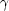s = 1. This is the case in external shocks, or in a shock between relativistic ejecta and a non-relativistic material that was ejected from the source before it exploded. Eqs. 39 reveal that a mass: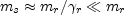(39)

is needed to yieldm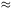r / 2 andmr / 2. The external mass needed to convert half of the kinetic energy is smaller than the original mass by a factor ofr [27, 18].

The second case corresponds to an internal collision between shells that are moving at different relativistic velocities:rs >> 1. Eqs. 39 yield: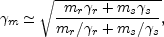(40)

The internal energy (in the frame of an external observer) of the merged shell, Eint =m, is the difference of the kinetic energies before and after the collision: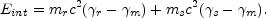(41)
The conversion efficiency of kinetic energy into internal energy is [33, 69]: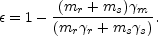(42)

As can be expected a conversion of a significant fraction of the initial kinetic energy to internal energy requires that the difference in velocities between the shells will be significant:r >>s and that the two masses will be comparable mrms.

Quite often the previous estimates based on the approximation that the whole shell behaves as a single object are good enough. However, the time scale between the interaction of different parts of the shell with the ISM may be relatively long (compared to the time scale to collect an external mass M /) and in this case one has to turn to the hydrodynamics of the interaction. This calculation takes into account the shocks that form during the collision.

Consider the situation a cold shell (whose internal energy is negligible compared to the rest mass) that overtakes another cold shell or moves into the cold ISM. Generally, Two shocks form: an outgoing shock that propagates into the ISM or into the external shell, and a reverse shock that propagates into the inner shell, with a contact discontinuity between the shocked material (see Fig. 21). Two quantities determine the shocks' structure:, the Lorentz factor of the motion of the inner shell (denoted 4) relative to the outer one - or the ISM (denoted 1) and the ratio between the particle number densities in these regions, fn4 / n1.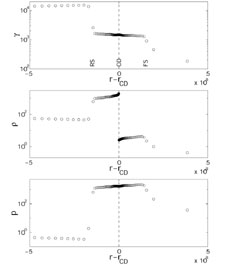Figure 21. The Lorentz factor, the densityand the pressure p in the shocks. There are four regions: the ISM (region 1), the shocked ISM (region 2), the shocked shell (region 3) and the unshocked shell (region 4), which are separated by the forward shock (FS), the contact discontinuity (CD) and the reverse shock (RS). The initial parameters are the same as in 13. From .

There are three interesting cases: (i) Ultra-relativistic shock (>> 1) with f >2. This happens during the early phase of an external shock or during the very late external shock evolution when there is only a single shock. We call this configuration "Newtonian" because the reverse shock is non-relativistic (or mildly relativistic). In this case the energy conversion takes place in the forward shock: Let2 be the Lorentz factor of the motion of the shocked fluid relative to the rest frame of the fluid at 1 (an external observer for interaction with the ISM and the outer shell in case of internal collision) and let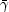3 be the Lorentz factor of the motion of this fluid relative to the rest frame of the relativistic shell (4).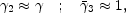(43)

The particle and energy densities (n, e) in the shocked regions satisfy: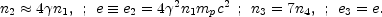(44)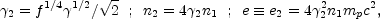(45)

Similar relations hold for the reverse shock: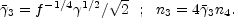(46)

In addition we have e3 = e and3(/2 +2 /) / 2 which follow from the equality of pressures and velocity on the contact discontinuity. Comparable amounts of energy are converted to thermal energy in both shocks when both shocks are relativistic. But only a negligible amount of energy is converted to thermal energy in the reverse shock if it is Newtonian .

(iii) Internal shocks are characterized by f1 - both shells have similar densities, and by a Lorentz factor of order of a few (2 << 10) describing the relative motion of the shells. In this case, for an adiabatic index (4/3) we have: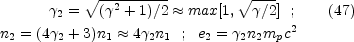(47)

Both shocks are mildly relativistic and their strength depends on the relative Lorentz factors of the two shells.

Before concluding this section and turning to the radiation mechanisms we summarize briefly the different relativistic motions encountered when considering different emitting regions. The relativistic shocks are characterized bysh that describes the shock's velocity as well as the "thermal" motion of the shocked particles. It is measured relative to a rest frame in which the unshocked material is at rest. The Lorentz factor of the forward shock is usually different from the Lorentz factor of the reverse shock. The emitting region - the shocked material - moves relativistically relative to an observer at rest at infinity. This is characterized by a Lorentz factor,E. Table 8.1.3 summarizes the different values ofsh andE for external and internal shocks and for the afterglow.

 Shock typeEsh External Newtonian ForwardReverse1 Relativistic Forward3/43/4 Reverse3/4-3/4 Internal(int / 2)1/2 ~ a few Afterglow(t)(t)